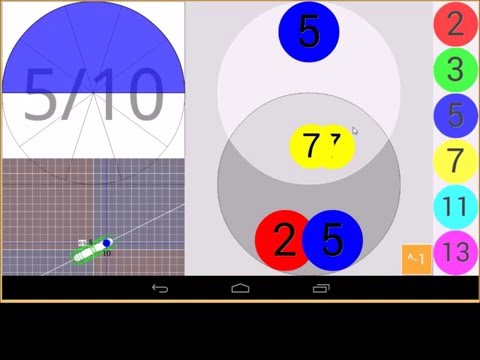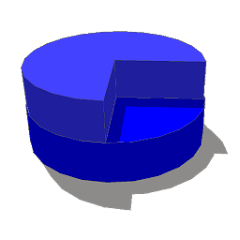# Touch Fraction ℚ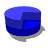2.6
22 reviews
1K+Everyone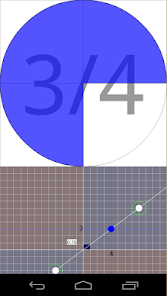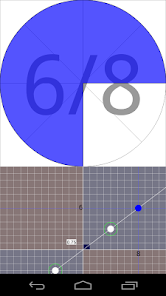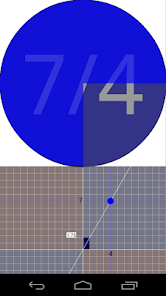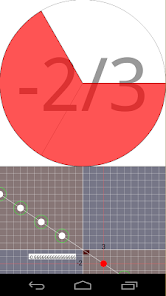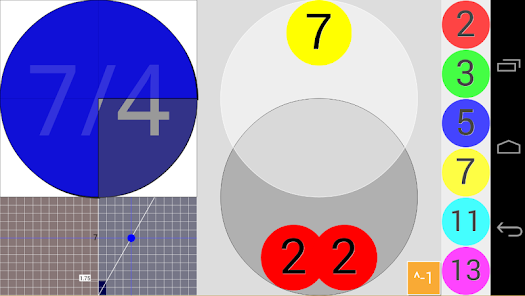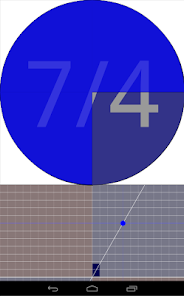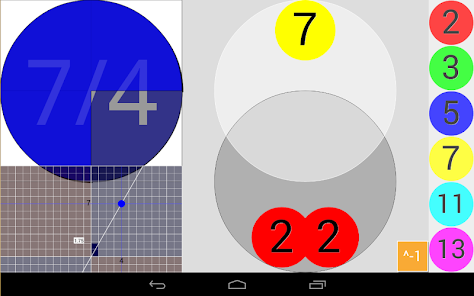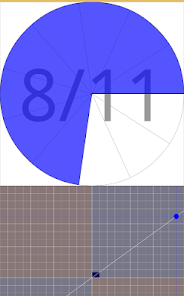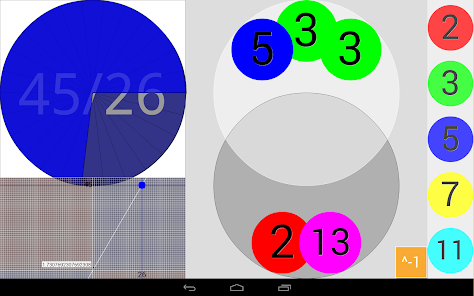Interactive fraction.
Fraction Sense
About fractions, and the construction of the rational numbers: ℚ set.
To play with proper and improper fractions, positive and negative also.

All fractions in order from least to greatest. You can increase or decrease the fractions included in the app, increasing or decreasing the density of lines in the lower graph framework. The accuracy of the application depends on the capabilities of the device.

The application provides two representations: a circle and a straight line whose has the slope of chosen fraction

Spin the fraction: You can choose different fractions in order from low to high within the possibilities included in the grid at the bottom formed by pairs of integers.
Select equivalent fractions with the rational representation diagram (the lower grid).
Change range of fractions affordable with a "pinch zoom" on the lower grid.

In the vertical strip of the grid graph goes from 0 to 1 you can see the number of units containing the selected improper fraction: Each unit is a black square.

In version 1.4.x has been added the prime factorization of the numerator and denominator, making it easier to visualize equivalent fractions. The program can also be controlled from the part of the prime factors.
At right there's the prime list of tokens to be used in the program.
The circles dedicated to the numerator and denominator circle, overlap:
To simplify fractions place prime tokens in the common area:
The multiplication of the common prime tokens is the Greatest Common Divisor (GCD)

Prime numbers are the basic building blocks of numbers:
This program uses the Fundamental Theorem of Arithmetic to simplify fractions or to build proper and improper fractions.

The app is useful to learn about fractions, coordinate geometry, rational numbers, cartesian plane, slope, prime factorization and the equivalent classes of pairs of integers.

And from version 1.4.x: http://nummolt.blogspot.com/2015/12/touch-fraction-14x.html
Touch Fraction ℚ is a complete tool to understand positive fractions, negative fractions, positive and negative numerators, positive and negative denominators, equivalent fractions, and inverted fraction.﻿
And for to teach about GCD (Greatest Common Divisor) to reduce fractions.

Nummolt construction toys:
http://www.nummolt.com
Nummolt apps: "Not Montessori per se, but Montessori-like"

MathTools reference:

Math 1, 2, 3, 4, 5 Fractions
Math 6 All previous and Rect coordinate geometry
Math 7 All previous and Rational numbers, Linear relationships, Ratio and proportion
Geometry Points and lines, Cartesian plane, Slope
Algebra Whole, Rational

Touch Fraction Q contains the foundations of fractions in Math Garden development. (same developer)
Updated on
Sep 13, 2022

## Data safety

Safety starts with understanding how developers collect and share your data. Data privacy and security practices may vary based on your use, region, and age. The developer provided this information and may update it over time.No data shared with third partiesNo data collected

## Ratings and reviews

3.0
17 reviews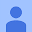September 25, 2018
U cant get certain fractions
1 person found this review helpful
nummolt
September 26, 2018
Touch Fraction shows the available fractions on the grid: Pinch Zoom on the lower grid. This will increase or decrease available fractions.